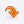UniversitiesLOGINCONTACTMost Recent ArticlesMost Rated ArticlesCategories

# Travel/ TourismGATE
Marking Schema in GATE 2013
Author : Kennice
 Category : GATE posted Date :
 Total No.of views : Total No.of Comments : 0
Rating:0 / 5 (0 votes)

Tags :
GATE Exam Pattern, Marking Schema

Marking Schema in GATE 2013Marking Scheme

For 1 mark multiple choice questions, 1/3 mark will be deducted for a wrong answer. Likewise, for 2 marks multiple choice questions, 2/3  mark will be deducted for a wrong answer. However, for the linked answer question pair, where each question carries 2 marks, 2/3  mark will be deducted for a wrong answer to the first question only. There is no negative marking for wrong answer to the second question of the linked answer question pair. If the first question in the linked pair is wrongly answered or is unattempted, then the answer to the second question in the pair will not be evaluated. There is no negative marking for numerical answer type questions (numerical answer type questions will appear only in the papers for which the exam is ONLINE only).

General Aptitude (GA) Questions

In all papers, GA questions are of multiple choice type, and carry a total of 15 marks.  The GA section includes 5 questions carrying 1 mark each (sub-total 5 marks) and 5 questions carrying 2 marks each (sub-total 10 marks).

Question papers other than GG, XE and XL

These papers would contain 25  questions carrying one mark each (sub-total 25 marks) and 30  questions carrying two marks each (sub-total 60 marks).  Out of these, two pairs of questions would be common data questions, and two pairs of questions would be linked answer questions.  In the ONLINE papers, the question paper will consist of  questions of multiple choice type and numerical answer type. For multiple choice type questions, each question will have four choices for the answer. For numerical answer type questions, each question will have a number as the answer and choices will not be givenThe answer is to be entered using the mouse and a virtual keypad that will appear on the screen.

GG (Geology and Geophysics) Paper

Apart from the General Aptitude (GA) section, the GG question paper consists of two parts: Part A and Part B. Part A is common for all candidates. Part B contains two sections: Section 1 (Geology) and Section 2 (Geo-physics). Candidates will have to attempt questions in Part A and either Section 1 or Section 2 in Part B.

Part A consists of 25 multiple choice questions carrying 1-mark each (sub-total 25 marks & some of these may be numerical questions). Each section  in Part B (Section 1 and Section 2) consists of 30 multiple choice questions carrying 2 marks each (sub-total 60 marks and some of these may be numerical questions). Out of these, two pairs of questions would be common data questions, and two pairs of questions would be linked answer questions.

XE Paper (Engineering Sciences)

In XE paper, Engineering Mathematics section (Section A) is compulsory. This section contains 11 multiple choice questions carrying a total of 15 marks: 7 questions carrying 1-mark each (sub-total 7 marks), and 4 questions carrying 2-marks each (sub-total 8 marks). Some of the multiple choice questions may be replaced by numerical questions.

Each of the other sections of the XE paper (Sections B through G) contains 22 questions carrying a total of 35 marks:  9 questions carrying 1 mark each (sub-total 9 marks) and 13 questions carrying 2 marks each (sub-total 26 marks).  Out of the 2 mark questions, 2 pairs are common data questions and 1 pair is linked answer questions. Some of the multiple choice questions may be replaced by numerical questions.

XL Paper (Life Sciences)

In XL paper,  Chemistry section (Section H) is compulsory. This section contains 15 multiple choice questions carrying a total of 25 marks: 5 questions carrying 1 mark each (sub-total 5 marks) and  10 questions carrying 2-marks each (sub-total 20 marks).  Out of the 2-mark questions, 1 pair is common data questions, and 1 pair is linked answer questions. Some of the multiple choice questions may be replaced by numerical questions.

Each of the other sections of the XL paper (Sections I through M) contains 20 multiple choice questions carrying a total of 30 marks: 10 questions carrying 1 mark each (sub-total 10 marks) and 10 questions carrying 2 marks each (sub-total 20 marks). Some of the multiple choice questions may be replaced by numerical questions.

## CommentsReceive All Updates Via Facebook